# Near zeros of zeta

Alex Kontorovich had an interesting tweet about the Riemann hypothesis a few days ago.

The critical strip of the Riemann zeta function is the portion of the complex plane with real part between 0 and 1. The Riemann hypothesis conjectures that the zeros of the zeta function in this region all have real part 1/2. To put it another way, the Riemann hypothesis says that ζ(x + it) is never zero unless x = 1/2. However, according to Alex Kontorovich’s tweet, the function gets arbitrarily close to zero infinitely often for other values of x.

I wrote a little Mathematica code to produce graphs like the ones above. Here is a plot of ζ(1/2 + it).

```    ParametricPlot[
{Re[Zeta[1/2 + I t]], Im[Zeta[1/2 + I t]]},
{t, 0, 100},
AspectRatio -> 1,
PlotRange -> {{-2, 6}, {-3, 3}}
]
```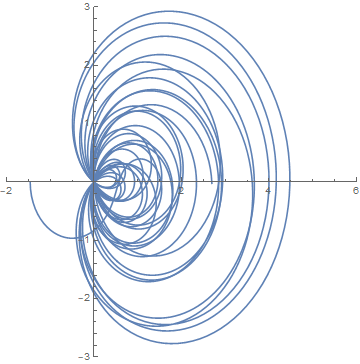At first I didn’t specify the aspect ratio and I got nearly circular arcs. Then I realized that was an artifact of Mathematica’s default aspect ratio. Also, I set the plot range so the image above would be comparable with the next image.

Notice all the lines crossing the origin. These correspond to the zeros of ζ along the line Re(x) = 1/2.

Next I changed the 1/2 to 1/3.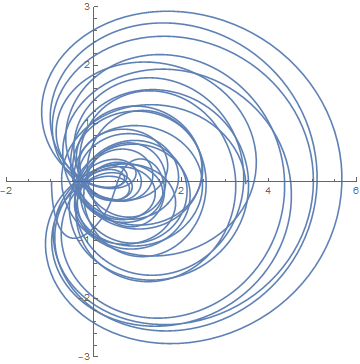If you look closely you’ll see a gap around the origin.

Here’s a closeup of the origin in both graphs. First for x = 1/2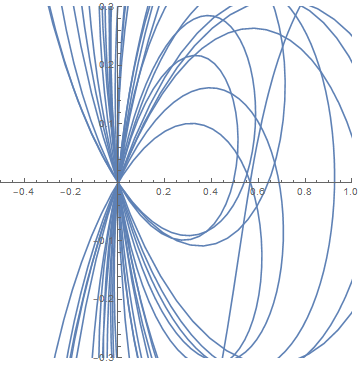and now for x = 1/3.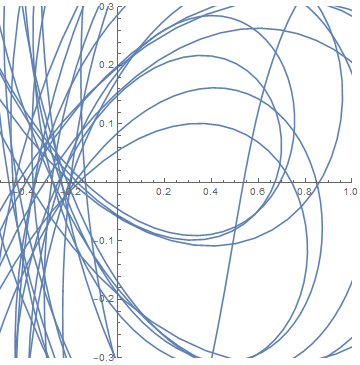I hadn’t seen the result in Kontorovich’s tweet before. I don’t know whether it holds for all x or just for certain values of x. I assume the former. But apparently it at least holds for x = 4/5. So we should be able to find values of |&zeta(4/5 + it)| as small as we like by taking t large enough, and we should always be able to find more such values by looking further out. In practice, you might have to very far out before you find a small value. Looking as far as 1000, for example, it seems the minimum is about 0.2.

```    Plot[
Abs[Zeta[4/5 + I t]],
{t, 0, 1000},
PlotRange -> {0, 1}
]
```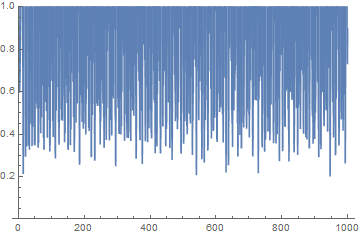If you ask Mathematica to find the minimum in the graph above, it won’t give a reasonable answer; there are too many local minima. If instead you ask it to look repeatedly over small intervals, it does much better. The following minimizes |ζ(4/5 + it)| over the interval [i, i+1].

```    f[i_] := FindMinimum[
{Abs[Zeta[4/5 + I t]], i <= t <= i + 1},
{t, i}
]
```

It returns a pair: the minimum and where it occurred. The following will look for places where |ζ(4/5 + it)| is less than 0.2.

```    For[i = 0, i < 1000, i++,
If[ First[f[i]] < 0.20,
Print[f[i]]
]
]
```

It finds two such values.

```{0.191185, {t->946.928}}

{0.195542, {t->947.}}
```

As I write this I have a longer program running, and so far the smallest value I’ve found is 0.1809 which occurs at t = 1329.13. According to Kontorovich you can find as small a value as you like, say less than 0.1 or 0.001, but apparently it might take a very long time.

Update: The minimum over 0 ≤ t ≤ 10,000 is 0.142734, which occurs at 7563.56.

## 5 thoughts on “Near zeros of zeta”

1. Sigh. I waited more than a day for a meaningful, contextual comment to appear before posting mine. So I could have something to hide behind (or below). But I can’t keep it in any longer.

The title of this post reads like the title of a SciFi pulp novel from the 1930’s, or perhaps the title of a B-movie from the 1950’s. Especially if you insert “The” as the first word. Go ahead: Read it again, and tell me I’m wrong.

Now, try remembering what John’s post was about. Surprisingly tough, right?

My work here is done. Thank you for your kind attention (and the diversion thereof).

2. I can imagine the cover art …

3. The fact that zeta(q+it) has infinitely many near zeros for any fixed 1/2 < q < 1, is a consequence of Voronin's Zeta Function Universality. This states any nowhere zero holomorphic function f(s) on a compact region C contained in the strip with real part 1/2<r<1, can be approximated arbitrarily well by zeta(s+it) for some t.

I think zeta function universality expresses just how difficult the Riemann Hypothesis is, even moreso than the near zero phenomenon.

4. Universality is a wild result. I had more confidence that RH was true before learning about universality. :)